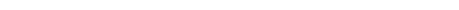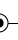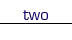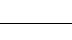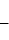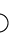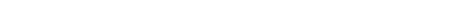In the Circle Rotation puzzles, you jump from circle to circle, following the black lines. The object of each puzzle is to get all of the black circle sections to point downward. In order to accomplish this goal, each time you move, the circle you land on will rotate according to the following chart (which is reprinted with each puzzle).There is a colored band around each circle, either red or green or blue. The rotation will depend upon the color of the circle you jump from as well as the color of the circle you jump to.

For example, say we wanted to start on the red circle below and jump to the green circle. According to the chart, the green circle would rotate four positions in the clockwise direction, thus the move would look like this: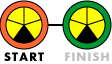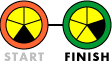However, say we wanted to instead start on the green circle and move to the red circle.

According to the chart, the red circle would rotate two positions in the clockwise direction, thus the move would now look like this: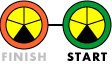For one final example, we'll move from the green circle to the blue circle to the red circle.

Moving from the green circle to the blue circle means that the blue circle is rotated four positions counterclockwise. Then moving from the blue circle to the red circle means that the red circle is rotated two positions counterclockwise.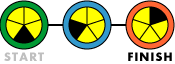Last updated: September 1, 2003